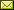## Qutrit Dichromatic Calculus and Its Universality

 Quanlong Wang (Beihang University) Xiaoning Bian (Beihang University)

 We introduce a dichromatic calculus (RG) for qutrit systems. We show that the decomposition of the qutrit Hadamard gate is non-unique and not derivable from the dichromatic calculus. As an application of the dichromatic calculus, we depict a quantum algorithm with a single qutrit. Since it is not easy to decompose an arbitrary d by d unitary matrix into Z and X phase gates when d > 2, the proof of the universality of qudit ZX calculus for quantum mechanics is far from trivial. We construct a counterexample to Ranchin's universality proof, and give another proof by Lie theory that the qudit ZX calculus contains all single qudit unitary transformations, which implies that qudit ZX calculus, with qutrit dichromatic calculus as a special case, is universal for quantum mechanics.

In Bob Coecke, Ichiro Hasuo and Prakash Panangaden: Proceedings of the 11th workshop on Quantum Physics and Logic (QPL 2014), Kyoto, Japan, 4-6th June 2014, Electronic Proceedings in Theoretical Computer Science 172, pp. 92–101.
Published: 28th December 2014.

 ArXived at: http://dx.doi.org/10.4204/EPTCS.172.7 bibtex PDF
References in reconstructed bibtex, XML and HTML format (approximated).
 Comments and questions to:eptcs@eptcs.org For website issues:webmaster@eptcs.org